## Graphs of Exponential Functions

### Learning Outcomes

• Determine whether an exponential function and its associated graph represents growth or decay.
• Sketch a graph of an exponential function.
• Graph exponential functions shifted horizontally or vertically and write the associated equation.
• Graph a stretched or compressed exponential function.
• Graph a reflected exponential function.
• Write the equation of an exponential function that has been transformed.

As we discussed in the previous section, exponential functions are used for many real-world applications such as finance, forensics, computer science, and most of the life sciences. Working with an equation that describes a real-world situation gives us a method for making predictions. Most of the time, however, the equation itself is not enough. We learn a lot about things by seeing their visual representations, and that is exactly why graphing exponential equations is a powerful tool. It gives us another layer of insight for predicting future events.

## Characteristics of Graphs of Exponential Functions

Before we begin graphing, it is helpful to review the behavior of exponential growth. Recall the table of values for a function of the form $f\left(x\right)={b}^{x}$ whose base is greater than one. We’ll use the function $f\left(x\right)={2}^{x}$. Observe how the output values in the table below change as the input increases by 1.

 x –3 –2 –1 0 1 2 3 $f\left(x\right)={2}^{x}$ $\frac{1}{8}$ $\frac{1}{4}$ $\frac{1}{2}$ 1 2 4 8

Each output value is the product of the previous output and the base, 2. We call the base 2 the constant ratio. In fact, for any exponential function with the form $f\left(x\right)=a{b}^{x}$, b is the constant ratio of the function. This means that as the input increases by 1, the output value will be the product of the base and the previous output, regardless of the value of a.

Notice from the table that:

• the output values are positive for all values of x
• as x increases, the output values increase without bound
• as x decreases, the output values grow smaller, approaching zero

The graph below shows the exponential growth function $f\left(x\right)={2}^{x}$.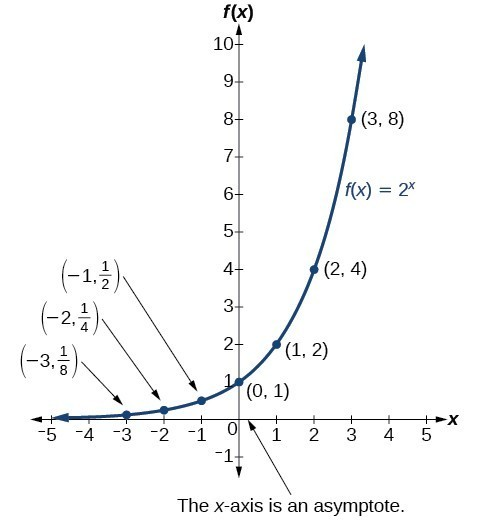Notice that the graph gets close to the x-axis but never touches it.

The domain of $f\left(x\right)={2}^{x}$ is all real numbers, the range is $\left(0,\infty \right)$, and the horizontal asymptote is $y=0$.

To get a sense of the behavior of exponential decay, we can create a table of values for a function of the form $f\left(x\right)={b}^{x}$ whose base is between zero and one. We’ll use the function $g\left(x\right)={\left(\frac{1}{2}\right)}^{x}$. Observe how the output values in the table below change as the input increases by 1.

 x –3 –2 –1 0 1 2 3 $g\left(x\right)=\left(\frac{1}{2}\right)^{x}$ 8 4 2 1 $\frac{1}{2}$ $\frac{1}{4}$ $\frac{1}{8}$

Again, because the input is increasing by 1, each output value is the product of the previous output and the base or constant ratio $\frac{1}{2}$.

Notice from the table that:

• the output values are positive for all values of x
• as x increases, the output values grow smaller, approaching zero
• as x decreases, the output values grow without bound

The graph below shows the exponential decay function, $g\left(x\right)={\left(\frac{1}{2}\right)}^{x}$.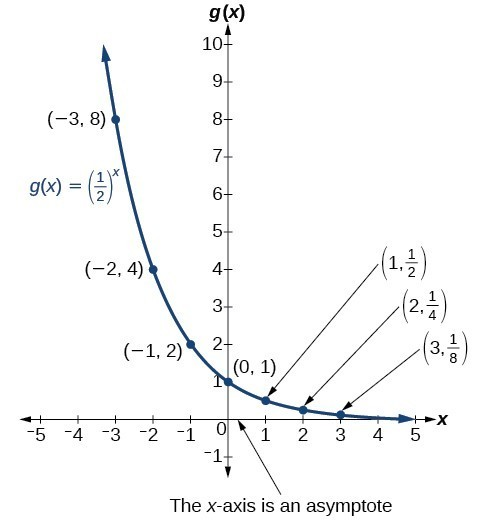The domain of $g\left(x\right)={\left(\frac{1}{2}\right)}^{x}$ is all real numbers, the range is $\left(0,\infty \right)$, and the horizontal asymptote is $y=0$.

### A General Note: Characteristics of the Graph of the Parent Function $f\left(x\right)={b}^{x}$

An exponential function with the form $f\left(x\right)={b}^{x}$, $b>0$, $b\ne 1$, has these characteristics:

• one-to-one function
• horizontal asymptote: $y=0$
• domain: $\left(-\infty , \infty \right)$
• range: $\left(0,\infty \right)$
• x-intercept: none
• y-intercept: $\left(0,1\right)$
• increasing if $b>1$
• decreasing if $b<1$

### How To: Given an exponential function of the form $f\left(x\right)={b}^{x}$, graph the function

1. Create a table of points.
2. Plot at least 3 point from the table including the y-intercept $\left(0,1\right)$.
3. Draw a smooth curve through the points.
4. State the domain, $\left(-\infty ,\infty \right)$, the range, $\left(0,\infty \right)$, and the horizontal asymptote, $y=0$.

### Example: Sketching the Graph of an Exponential Function of the Form $f\left(x\right)={b}^{x}$

Sketch a graph of $f\left(x\right)={0.25}^{x}$. State the domain, range, and asymptote.

### Try It

Sketch the graph of $f\left(x\right)={4}^{x}$. State the domain, range, and asymptote.

## Graphing Exponential Functions Using Transformations

Transformations of exponential graphs behave similarly to those of other functions. Just as with other parent functions, we can apply the four types of transformations—shifts, reflections, stretches, and compressions—to the parent function $f\left(x\right)={b}^{x}$ without loss of shape. For instance, just as the quadratic function maintains its parabolic shape when shifted, reflected, stretched, or compressed, the exponential function also maintains its general shape regardless of the transformations applied.

### Graphing a Vertical Shift

The first transformation occurs when we add a constant d to the parent function $f\left(x\right)={b}^{x}$ giving us a vertical shift d units in the same direction as the sign. For example, if we begin by graphing a parent function, $f\left(x\right)={2}^{x}$, we can then graph two vertical shifts alongside it using $d=3$: the upward shift, $g\left(x\right)={2}^{x}+3$ and the downward shift, $h\left(x\right)={2}^{x}-3$. Both vertical shifts are shown in the figure below.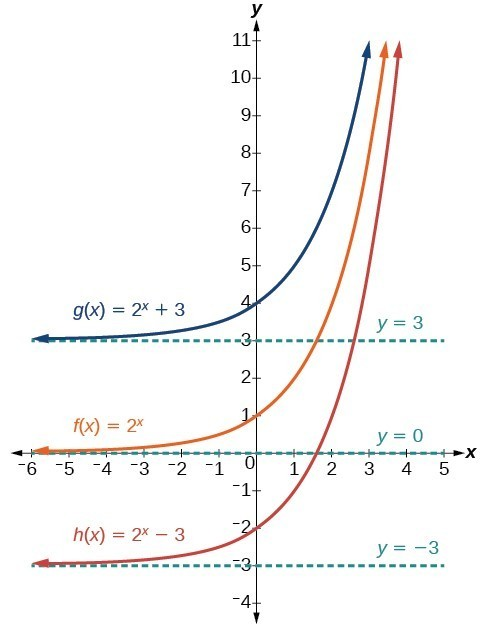Observe the results of shifting $f\left(x\right)={2}^{x}$ vertically:

• The domain $\left(-\infty ,\infty \right)$ remains unchanged.
• When the function is shifted up 3 units giving $g\left(x\right)={2}^{x}+3$:
• The y-intercept shifts up 3 units to $\left(0,4\right)$.
• The asymptote shifts up 3 units to $y=3$.
• The range becomes $\left(3,\infty \right)$.
• When the function is shifted down 3 units giving $h\left(x\right)={2}^{x}-3$:
• The y-intercept shifts down 3 units to $\left(0,-2\right)$.
• The asymptote also shifts down 3 units to $y=-3$.
• The range becomes $\left(-3,\infty \right)$.

### Graphing a Horizontal Shift

The next transformation occurs when we add a constant c to the input of the parent function $f\left(x\right)={b}^{x}$ giving us a horizontal shift c units in the opposite direction of the sign. For example, if we begin by graphing the parent function $f\left(x\right)={2}^{x}$, we can then graph two horizontal shifts alongside it using $c=3$: the shift left, $g\left(x\right)={2}^{x+3}$, and the shift right, $h\left(x\right)={2}^{x - 3}$. Both horizontal shifts are shown in the graph below.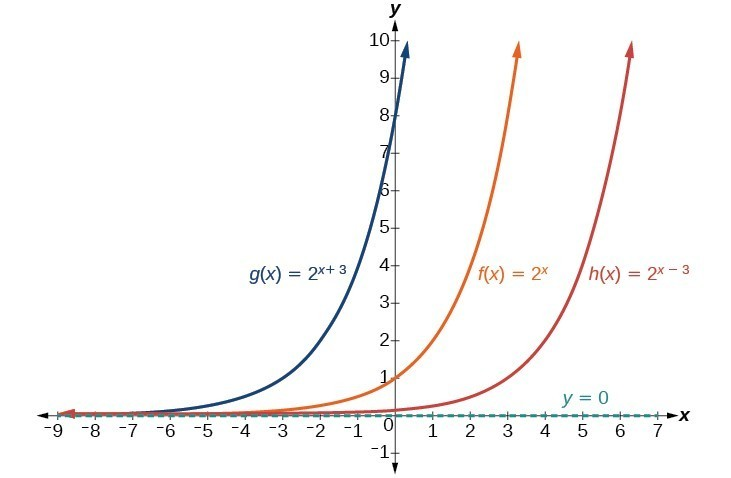Observe the results of shifting $f\left(x\right)={2}^{x}$ horizontally:

• The domain, $\left(-\infty ,\infty \right)$, remains unchanged.
• The asymptote, $y=0$, remains unchanged.
• The y-intercept shifts such that:
• When the function is shifted left 3 units to $g\left(x\right)={2}^{x+3}$, the y-intercept becomes $\left(0,8\right)$. This is because ${2}^{x+3}=\left({2}^{3}\right){2}^{x}=\left(8\right){2}^{x}$, so the initial value of the function is 8.
• When the function is shifted right 3 units to $h\left(x\right)={2}^{x - 3}$, the y-intercept becomes $\left(0,\frac{1}{8}\right)$. Again, see that ${2}^{x-3}=\left({2}^{-3}\right){2}^{x}=\left(\frac{1}{8}\right){2}^{x}$, so the initial value of the function is $\frac{1}{8}$.

### A General Note: Shifts of the Parent Function $f\left(x\right)={b}^{x}$

For any constants c and d, the function $f\left(x\right)={b}^{x+c}+d$ shifts the parent function $f\left(x\right)={b}^{x}$

• shifts the parent function $f\left(x\right)={b}^{x}$ vertically d units, in the same direction as the sign of d.
• shifts the parent function $f\left(x\right)={b}^{x}$ horizontally c units, in the opposite direction as the sign of c.
• has a y-intercept of $\left(0,{b}^{c}+d\right)$.
• has a horizontal asymptote of yd.
• has a range of $\left(d,\infty \right)$.
• has a domain of $\left(-\infty ,\infty \right)$ which remains unchanged.

### How To: Given an exponential function with the form $f\left(x\right)={b}^{x+c}+d$, graph the translation

1. Draw the horizontal asymptote yd.
2. Shift the graph of $f\left(x\right)={b}^{x}$ left c units if c is positive and right $c$ units if c is negative.
3. Shift the graph of $f\left(x\right)={b}^{x}$ up d units if d is positive and down d units if d is negative.
4. State the domain, $\left(-\infty ,\infty \right)$, the range, $\left(d,\infty \right)$, and the horizontal asymptote $y=d$.

### Example: Graphing a Shift of an Exponential Function

Graph $f\left(x\right)={2}^{x+1}-3$. State the domain, range, and asymptote.

In the following video, we show more examples of the difference between horizontal and vertical shifts of exponential functions and the resulting graphs and equations.

### Stretching, Compressing, or Reflecting an Exponential Function

While horizontal and vertical shifts involve adding constants to the input or to the function itself, a stretch or compression occurs when we multiply the parent function $f\left(x\right)={b}^{x}$ by a constant $|a|>0$. For example, if we begin by graphing the parent function $f\left(x\right)={2}^{x}$, we can then graph the stretch, using $a=3$, to get $g\left(x\right)=3{\left(2\right)}^{x}$ and the compression, using $a=\frac{1}{3}$, to get $h\left(x\right)=\frac{1}{3}{\left(2\right)}^{x}$.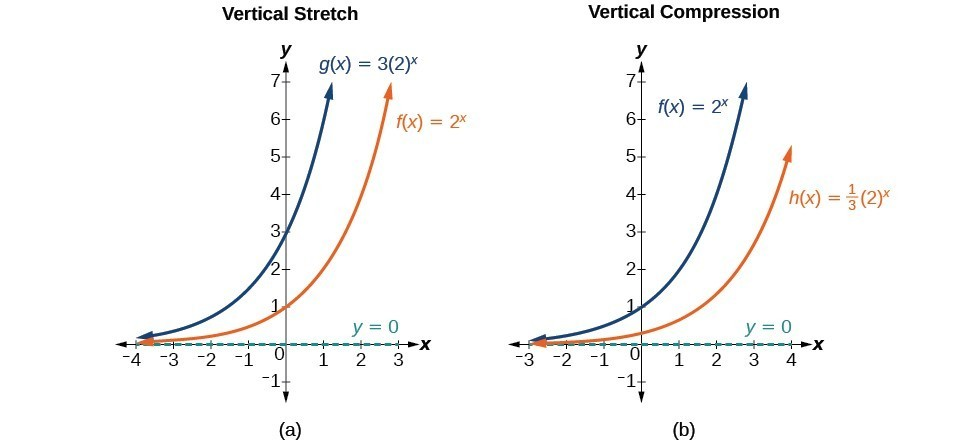(a) $g\left(x\right)=3{\left(2\right)}^{x}$ stretches the graph of $f\left(x\right)={2}^{x}$ vertically by a factor of 3. (b) $h\left(x\right)=\frac{1}{3}{\left(2\right)}^{x}$ compresses the graph of $f\left(x\right)={2}^{x}$ vertically by a factor of $\frac{1}{3}$.

### A General Note: Stretches and Compressions of the Parent Function $f\left(x\right)={b}^{x}$

The function $f\left(x\right)=a{b}^{x}$

• is stretched vertically by a factor of if $|a|>1$.
• is compressed vertically by a factor of a if $|a|<1$.
• has a y-intercept is $\left(0,a\right)$.
• has a horizontal asymptote of $y=0$, range of $\left(0,\infty \right)$, and domain of $\left(-\infty ,\infty \right)$ which are all unchanged from the parent function.

### Example: Graphing the Stretch of an Exponential Function

Sketch a graph of $f\left(x\right)=4{\left(\frac{1}{2}\right)}^{x}$. State the domain, range, and asymptote.

### Try It

Sketch the graph of $f\left(x\right)=\frac{1}{2}{\left(4\right)}^{x}$. State the domain, range, and asymptote.

### Graphing Reflections

In addition to shifting, compressing, and stretching a graph, we can also reflect it about the x-axis or the y-axis. When we multiply the parent function $f\left(x\right)={b}^{x}$ by –1, we get a reflection about the x-axis. When we multiply the input by –1, we get a reflection about the y-axis. For example, if we begin by graphing the parent function $f\left(x\right)={2}^{x}$, we can then graph the two reflections alongside it. The reflection about the x-axis, $g\left(x\right)={-2}^{x}$, and the reflection about the y-axis, $h\left(x\right)={2}^{-x}$, are both shown below.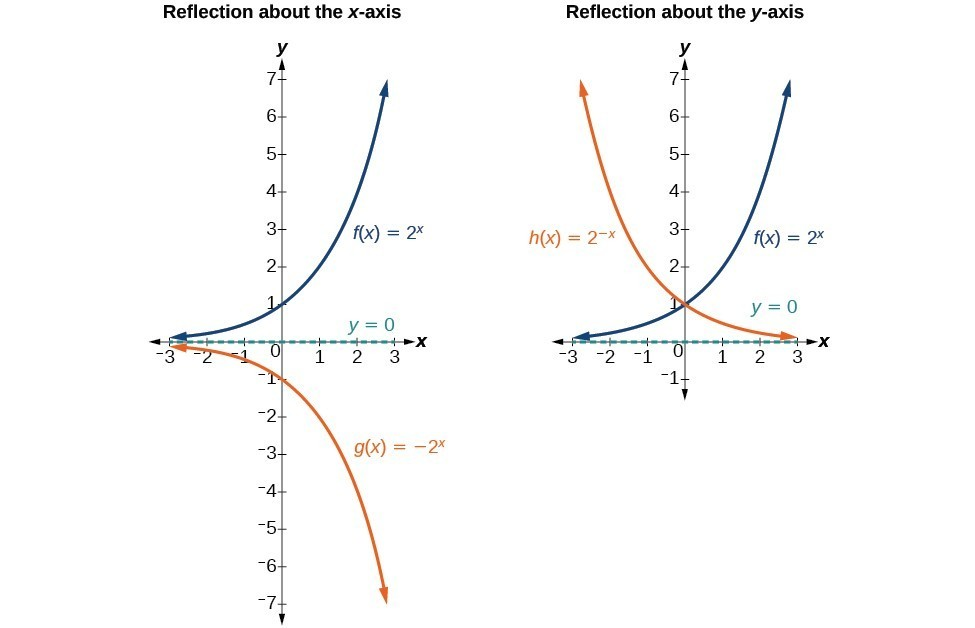(a) $g\left(x\right)=-{2}^{x}$ reflects the graph of $f\left(x\right)={2}^{x}$ about the x-axis. (b) $h\left(x\right)={2}^{-x}$ reflects the graph of $f\left(x\right)={2}^{x}$ about the y-axis.

### A General Note: Reflecting the Parent Function $f\left(x\right)={b}^{x}$

The function $f\left(x\right)=-{b}^{x}$

• reflects the parent function $f\left(x\right)={b}^{x}$ about the x-axis.
• has a y-intercept of $\left(0,-1\right)$.
• has a range of $\left(-\infty ,0\right)$.
• has a horizontal asymptote of $y=0$ and domain of $\left(-\infty ,\infty \right)$ which are unchanged from the parent function.

The function $f\left(x\right)={b}^{-x}$

• reflects the parent function $f\left(x\right)={b}^{x}$ about the y-axis.
• has a y-intercept of $\left(0,1\right)$, a horizontal asymptote at $y=0$, a range of $\left(0,\infty \right)$, and a domain of $\left(-\infty ,\infty \right)$ which are unchanged from the parent function.

### Example: Writing and Graphing the Reflection of an Exponential Function

Find and graph the equation for a function, $g\left(x\right)$, that reflects $f\left(x\right)={\left(\frac{1}{4}\right)}^{x}$ about the x-axis. State its domain, range, and asymptote.

## Summarizing Transformations of the Exponential Function

Now that we have worked with each type of translation for the exponential function, we can summarize them to arrive at the general equation for transforming exponential functions.

Transformations of the Parent Function $f\left(x\right)={b}^{x}$
Transformation Form
Shift

• Horizontally c units to the left
• Vertically d units up
$f\left(x\right)={b}^{x+c}+d$
Stretch and Compression

• Stretch if |a|>1
• Compression if 0<|a|<1
$f\left(x\right)=a{b}^{x}$
Reflection about the x-axis $f\left(x\right)=-{b}^{x}$
Reflection about the y-axis $f\left(x\right)={b}^{-x}={\left(\frac{1}{b}\right)}^{x}$
General equation for all transformations $f\left(x\right)=a{b}^{x+c}+d$

### A General Note: Transformations of Exponential Functions

A transformation of an exponential function has the form

$f\left(x\right)=a{b}^{x+c}+d$, where the parent function, $y={b}^{x}$, $b>1$, is

• shifted horizontally c units to the left.
• stretched vertically by a factor of $|a|$ if $|a| > 1$.
• compressed vertically by a factor of $|a|$ if $0 < |a| < 1$.
• shifted vertically d units.
• reflected about the x-axis when < 0.

Note the order of the shifts, transformations, and reflections follow the order of operations.

### Example: Writing a Function from a Description

Write the equation for the function described below. Give the horizontal asymptote, domain, and range.

• $f\left(x\right)={e}^{x}$ is vertically stretched by a factor of 2, reflected across the y-axis, and then shifted up 4 units.

### Try It

Write the equation for the function described below. Give the horizontal asymptote, the domain, and the range.

• $f\left(x\right)={e}^{x}$ is compressed vertically by a factor of $\frac{1}{3}$, reflected across the x-axis, and then shifted down 2 units.

## Using a Graph to Approximate a Solution to an Exponential Equation

Graphing can help you confirm or find the solution to an exponential equation. For example,$42=1.2{\left(5\right)}^{x}+2.8$ can be solved to find the specific value for x that makes it a true statement. Graphing $y=4$ along with $y=2^{x}$ in the same window, the point(s) of intersection if any represent the solutions of the equation.

To use a calculator to solve this, press [Y=] and enter $1.2(5)x+2.8$ next to Y1=. Then enter 42 next to Y2=. For a window, use the values –3 to 3 for$x$ and –5 to 55 for$y$.Press [GRAPH]. The graphs should intersect somewhere near$x=2$.

For a better approximation, press [2ND] then [CALC]. Select [5: intersect] and press [ENTER] three times. The x-coordinate of the point of intersection is displayed as 2.1661943. (Your answer may be different if you use a different window or use a different value for Guess?) To the nearest thousandth,x≈2.166.

### Try It

Solve $4=7.85{\left(1.15\right)}^{x}-2.27$ graphically. Round to the nearest thousandth.

## Key Equations

 General Form for the Transformation of the Parent Function $\text{ }f\left(x\right)={b}^{x}$ $f\left(x\right)=a{b}^{x+c}+d$

## Key Concepts

• The graph of the function $f\left(x\right)={b}^{x}$ has a y-intercept at $\left(0, 1\right)$, domain of $\left(-\infty , \infty \right)$, range of $\left(0, \infty \right)$, and horizontal asymptote of $y=0$.
• If $b>1$, the function is increasing. The left tail of the graph will approach the asymptote $y=0$, and the right tail will increase without bound.
• If 0 < b < 1, the function is decreasing. The left tail of the graph will increase without bound, and the right tail will approach the asymptote $y=0$.
• The equation $f\left(x\right)={b}^{x}+d$ represents a vertical shift of the parent function $f\left(x\right)={b}^{x}$.
• The equation $f\left(x\right)={b}^{x+c}$ represents a horizontal shift of the parent function $f\left(x\right)={b}^{x}$.
• The equation $f\left(x\right)=a{b}^{x}$, where $a>0$, represents a vertical stretch if $|a|>1$ or compression if $0<|a|<1$ of the parent function $f\left(x\right)={b}^{x}$.
• When the parent function $f\left(x\right)={b}^{x}$ is multiplied by –1, the result, $f\left(x\right)=-{b}^{x}$, is a reflection about the x-axis. When the input is multiplied by –1, the result, $f\left(x\right)={b}^{-x}$, is a reflection about the y-axis.
• All transformations of the exponential function can be summarized by the general equation $f\left(x\right)=a{b}^{x+c}+d$.
• Using the general equation $f\left(x\right)=a{b}^{x+c}+d$, we can write the equation of a function given its description.
• Approximate solutions of the equation $f\left(x\right)={b}^{x+c}+d$ can be found using a graphing calculator.